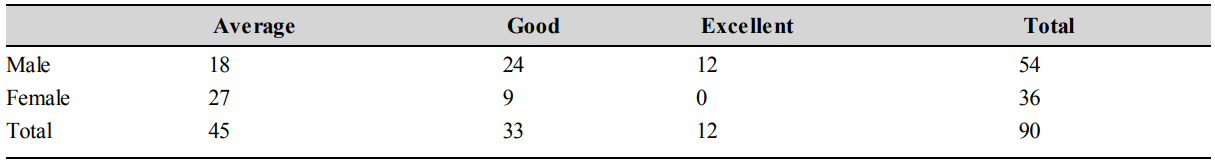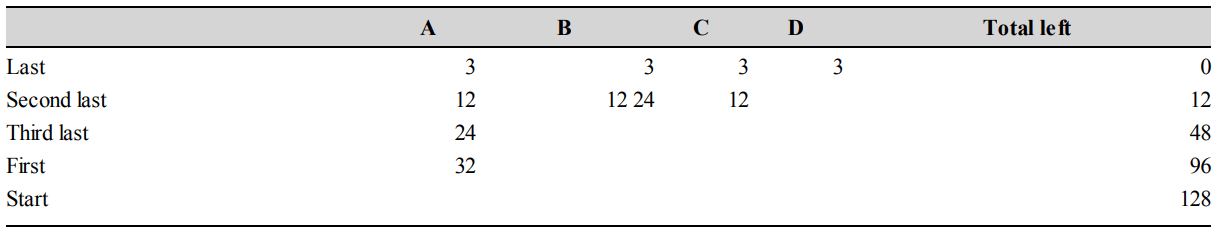## Quantitative Reasoning Questions and Answers Part-1

Data for Questions 1 to 5 : A quiz competition was organised in a school and the performance of students was recorded on piece of paper with ink. But somehow some water fell on the paper and the information remained incomplete
However the scorer has same clues which are:(i) Half the students were either excellent or good.
(ii) 40% of the students were females.
(iii) One-third of the males students were average.

1. How many students are both female and excellent?
a) 0
b) 8
c) 16
d) None of these

Explanation: From Clue No. (iii),
40% of the total students are female
40% = 36
100% = 90
Total students = 90Half of the students are either good or excellent means that total of (good + excellent) students = 45
\ Number of excellent = 12
1/3 of male = average male students = 18
The answer is 0. Option (a) is correct

2. What proportion of good students are male?
a) 0.73
b) 0
c) 0.4
d) None of these

Explanation: The answer is 0.73. Option (a) is correct (Refer explanation of Question 1)

3. What proportion of female students are good?
a) 0.25
b) 0
c) 5
d) None of these

Explanation: The answer is 0.25. Option (a) is correct (Refer explanation of Question 1)

4. How many students are both male and good?
a) 16
b) 24
c) 27
d) None of these

Explanation: The answer is 24. Option (b) is correct (Refer explanation of Question 1)

5. Among average students, what is the ratio of males to females?
a) 1:3
b) 2:3
c) 3:2
d) None of these

Explanation: The answer is 2:3. Option (b) is correct (Refer explanation of Question 1)

Data for Questions 6 to 10 : A, B, C and D are four friends living together in a flat and they have an agreement that whatever edible comes they will share equally among themselves. One day A’s uncle came to him and gave him a box of laddoos. Since no one was around, A divided the laddoos in four equal parts and ate his share after which he put the rest in the box. As he was closing the box, B walked in, took the Box from A & divided the ladoos in 4 equal parts & A & B took one part each and ate it. Suddenly C appeared and snatched the box. He again divided the laddoos in four equal parts, the three of them ate one part each and kept the remaining laddoos in the box. Later when D came he again divided the laddoos in four equal parts and all four ate their respective share. In total D ate 3 laddoos.

6. How many laddoos, in total did C eat?
a) 12
b) 15
c) 36
d) None of these

Explanation:3 + 12 = 15
Option (b) is correct

7. How many laddoos, in total did B eat?
a) 24
b) 15
c) 39
d) None of these

Explanation: 3 + 12 + 24 = 39
Option (c) is correct (Refer explanation of Question 6)

8. How many laddoos, in total did A eat?
a) 56
b) 68
c) 71
d) None of these

Explanation: 3 + 12 + 28 + 32 = 71
Option (c) is correct (Refer explanation of Question 6)

9. How many laddoos were given to A by his Uncle?
a) 128
b) 125
c) 113
d) None of these